## 2.18Heat capacity

When the heat conduction model of Eq. (2.54 ) is substituted into Eq. (2.51 ), we produce an equation with two variables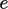and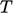. For example, starting with Eq. (2.56 ) for internal energy, and ignoring mechanical power and heat source terms, gives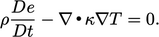(2.60)
To be able to solve this equation, a relation betweenandis needed. Heat capacity provides this, describing an amount of heat required to produce a unit change in. We use speciﬁc heat capacity, relating to a constant volume process, whose SI units are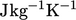, deﬁned as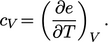(2.61)
Statistical mechanics provides a theorem known as equipartition which provides quantitative predictions of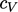.19 It calculates that any degree of freedom (DoF), such as a component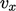of particle translational velocity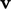, which appears quadratically in energy, i.e. as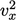, contributes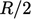to.

For monatomic gases, e.g. argon Ar, particle motion is translational only (until atoms dissociate into subatomic particles). With 3 translational DoFs,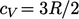is constant.

A gas which obeys the ideal gas law with constant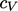is known as calorically perfect.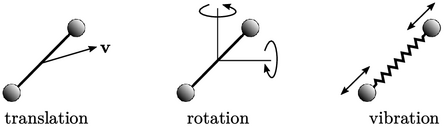For diatomic gases, e.g. nitrogen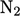, molecular motion is translational (left) and rotational (centre) at lower temperature (in the gas state). At higher temperature, vibrational motion is excited along its bond (right). Heat capacity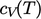for diatomic gases are then a function of temperature as shown below for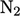.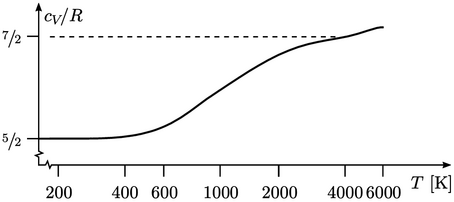Rotational motion provides 2 DoFs (rotational energy about the axis is negligible), giving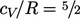. Beyond 500K, vibrational motion provides 2 additional DoFs (one kinetic, one potential), causing a gradual transition to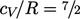. At high temperature, molecules dissociate and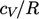increases further.

A thermally perfect gas is an ideal gas with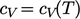. Often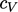can be treated as constant over a range of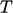, e.g.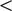600K for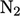. Otherwise, accurate calculations require a suitable model of. An imperfect gas exhibits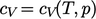.

19originating from heat capacity studies by Alexis Petit and Pierre Louis Dulong, Recherches sur quelques points importants de la théorie de la chaleur, 1819.

Notes on CFD: General Principles - 2.18 Heat capacity